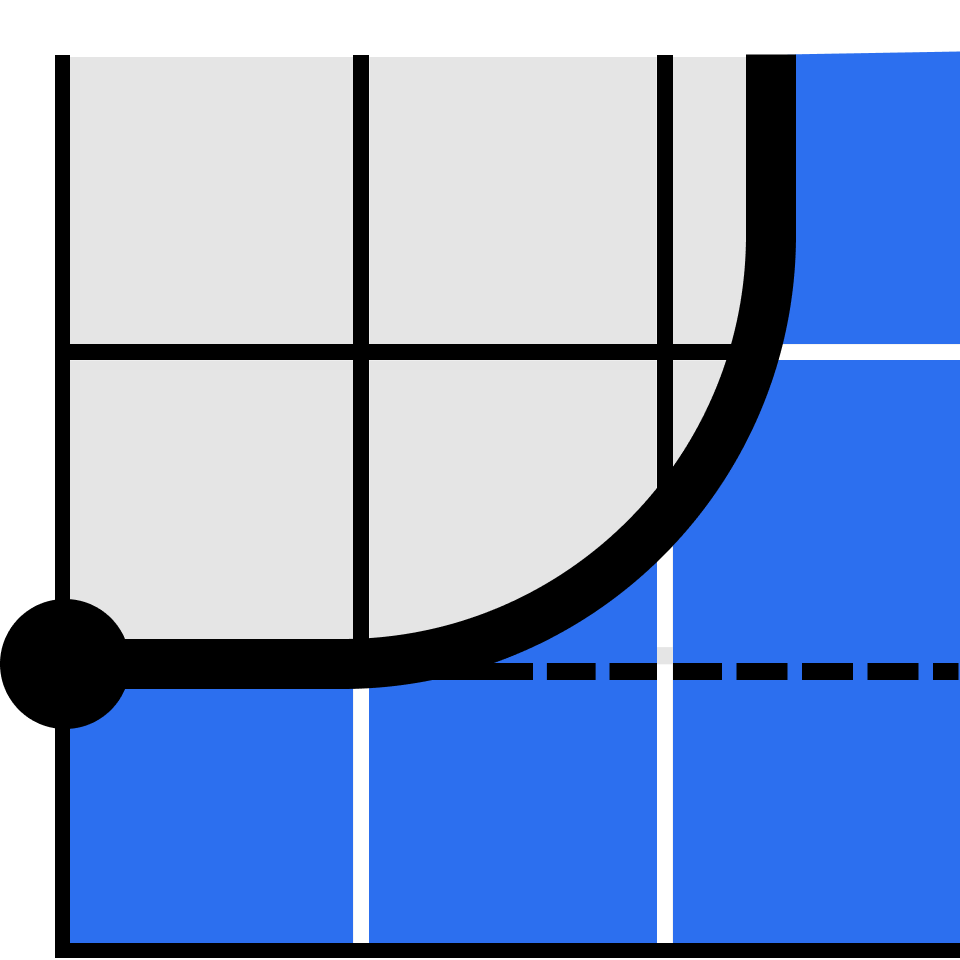Foundational Math · Level 2

## 2.1 Understanding Graphs

Supercharge your thinking with the language of algebra.

Seeing Solutions

Practice Seeing Solutions

Revealing Patterns

Practice Revealing Patterns

Graphing Equations

Practice Graphing Equations

Diminishing Returns

Intercepts

Practice Intercepts

Up and Down

Practice Up and Down

Minimums and Maximums

Practice Minimums and Maximums

Graph Gallery

Practice Graph Gallery

Optimizing Revenue

Steepness

Practice Steepness

The Y-Intercept

Practice The Y-Intercept

Parameters

Practice Parameters

Equations and Lines

Practice Equations and Lines

Problem Solving with Lines

Practice Problem Solving with Lines

Multiplying Equations

Practice Multiplying Equations

Parabola Features

Practice Parabola Features

Shaping Parabolas

Practice Shaping Parabolas

### Course description

This course explores the twin pillars of algebraic thinking—equations and graphs. With these two tools, we'll unpack algebra's big ideas and develop a powerful perspective to solve its essential problems. By the end of this course, you'll understand graphs and their relationship to the equations they represent, enabling you to answer questions involving equations even when it's impossible to solve them by hand. Though a familiarity with solving equations is helpful, we'll start with a review of the basics and build on them throughout the course. When you finish, you'll be ready to conquer a wide range of algebraic techniques in Algebra 1.

### Topics covered

• Algebraic Expressions
• Coordinate Plane
• Equations
• Graphs
• Linear Equations
• Ordered Pairs
• Rates
• Simplifying
• Systems of Equations
• Variables

### Prerequisites and next steps

Though a familiarity with solving equations is helpful, we'll start with a review of the basics and build on them throughout the course.

Up next

Foundational Math · Level 2

### 2.2 Measurement

Start exploring geometry with an intuitive introduction to the essentials.